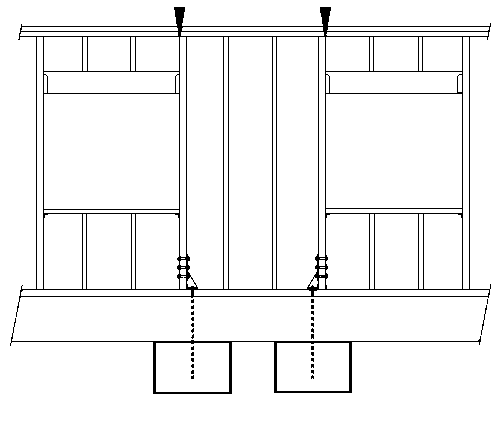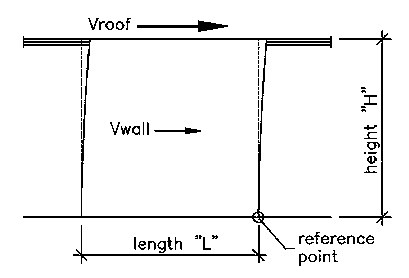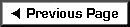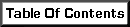A Residence Wall Resisting Seismic LoadingWall Information: Vroof = 800 pounds.  The weight of wall is 7 psf.  A roof contributes 350 plf to the top of this wall. The seismic coefficient is 0.183 and we are located in Zone 4.  Height = 8 feet. Width = 4 feet. The wall is framed from 2 x 4 Douglas Fir No. 1 except as required. The footing is 16" wide and 18" deep concrete. For simplicity, assume each header is 4 foot long.

 A Wall Between Headers To Resist Seismic Loading• In Zone 4, wood shear walls are limited by a 2:1 ratio of height to width.  This wall qualifies as a shear wall, ok.
• The total weight of the wall is (8)(4)(7 psf) = 224 pounds.
• The seismic force in the wall (Vwall) is (224)(0.183) = 40.99 pounds > Use 41 pounds.
• The overturning moment is (800)(8) + (41)(4) = 6564 ft-lbs.
• The resisting moment is in two parts. The first part is based on the wall weight and the roof load times half the wall width equal to (224 + (4)(350)) * (4/2) = 3248 ft-lbs.  The second part comes from the reaction of the far header time the distance from the near end of the wall equal to (2 * 350) * 4 = 2800 ft-llbs.  Total resisting moment is 6048 ft-lbs not countin gthe weight of window sills and headers above windows.  Very close.
• Total weight of wall plus tributary roof = (224 + (2+4)*350) = 2324 pounds.
• Since the overturning moment exceeds the resisting moment, a hold-down is required.
• The total unit shear in the wall is (800 + 41) / 4 = 210.25 lbs./ft.

Checking for compression posts and hold-downs...

Compression posts

• The end studs are going to be braced by sheathing for their entire length in the weak direction, therefore L/D = 96" / 3.5" = 27.42 (< 50, ok)
• Fc = (Cf for 4" stud width) * (Fc) = 1.15 * 1450 = 1667 psi
• Fce = (0.30* 1,700,000)/SQR(27.42) = 678 psi
• c = 0.80 for sawn lumber, so
• A = (1/1.6)*(1 + Fce/Fc) = 0.879
• B = (1/0.8)*(Fce/Fc) = 0.508
• Cp = A - SQRT( A*A - B) = 0.364
• Allowable unit load = (Cp) * (Fc) = (0.364) * (1667) = 606 psi (< Fc-perp. = 625 psi, ok).
• Total allowable load not to exceed (1.5) * (3.5) * (606) = 3,181 pounds.  Note this is more capacity than the weight of the whole roof plus wall system even without the 1.33 increase in stresses.

Hold-downs
Because we can already see that the hold-down force is going to be low, we can start by assuming a minimum hold-down capacity of 1000 pounds (the minimum for listed hold-down anchors) and do a much simpler calculation...

• Mot = (Vroof * H) + (Vwall * H/2) = 6,564 ft-lbs.
• Mr = (Calculated Mr based on weights) + (Hold-down Capacity)*(L - 0.5 ft) = (3248 + 2800 + 3500) = 9548 ft-lbs.
• The total weight of the system is (Wt. of Wall and Roof + Tiedown Force) = (2324 + 1000) = 3324 pounds.
• The distance to the centroid of compression is "a" which equals (Mr - Mot)/(Total Weight) = 0.89 feet.
• The maximum compression at the end of the wall is (Total Weight / a)*(2/3) = 2490 pounds.

End Stud Check
The distance "a" (see previous diagrams) indicates the distance to the centroid of compression forces.  (3 * a) is 2.67 feet.  The load on the end stud is (1245)*(1/2)*(16/12) + (2/3)*(1245)*(16/12)*(1/2) = 1383 pounds...plus the load from its header...for 2083 pounds total.  The adjacent stud would resist at most (3324 - 1383) = 1941 pounds.  Both are within the calculated limits, ok.

Shear and Sheathing
Our unit shear in this wall is 210.25 lbs./ft.  2x members are sufficient for framing all around.

The thickness of sheathing was not specified in the beginning, so we are left to select that as well as the nailing. Looking at Table 23-II-I-1 on page 2-288 of the 1997 UBC, we see our choices are:

• Structural I, 3/8" thick, with 8d nails at 6" o.c. at panel edges, 12" o.c. in the field, for 230 plf.
• C-D, C-C Sheathing, 3/8" thick, with 8d's at 6" o.c. at panel edges, 12" o.c. in the field, for 220 plf.

Sill Bolting
Looking at Table 23-III-B-1 on page 2-296, we see that the value for 1/2" diameter bolts in 2x material parallel to grain is 480 pounds.  With a 1.33 increase, this makes 638 pounds..

4 feet * 210.25 = 841 lbs.

(841) / (638) = 1.32 bolts > Use two 1/2" diameter bolts.  Space them at 16" centers near the interior of the wall to leave room for the hold-down bolts at the ends.